Q-Chem 5.0 User’s Manual

# 9.1 Equilibrium Geometries and Transition-State Structures

## 9.1.1 Overview

Molecular potential energy surfaces rely on the Born-Oppenheimer separation of nuclear and electronic motion. Minima on such energy surfaces correspond to the classical picture of equilibrium geometries, and transition state structures correspond to first-order saddle points. Both equilibrium and transition-state structures are stationary points for which the energy gradient vanishes. Characterization of such critical points requires consideration of the eigenvalues of the Hessian (second derivative matrix): minimum-energy, equilibrium geometries possess Hessians whose eigenvalues are all positive, whereas transition-state structures are defined by a Hessian with precisely one negative eigenvalue. (The latter is therefore a local maximum along the reaction path between minimum-energy reactant and product structures, but a minimum in all directions perpendicular to this reaction path.

The quality of a geometry optimization algorithm is of major importance; even the fastest integral code in the world will be useless if combined with an inefficient optimization algorithm that requires excessive numbers of steps to converge. Q-Chem incorporates a geometry optimization package (Optimize—see Appendix A) developed by the late Jon Baker over more than ten years.

The key to optimizing a molecular geometry successfully is to proceed from the starting geometry to the final geometry in as few steps as possible. Four factors influence the path and number of steps:

• starting geometry

• optimization algorithm

• quality of the Hessian (and gradient)

• coordinate system

Q-Chem controls the last three of these, but the starting geometry is solely determined by the user, and the closer it is to the converged geometry, the fewer optimization steps will be required. Decisions regarding the optimization algorithm and the coordinate system are generally made by the Optimize package (i.e., internally, within Q-Chem) to maximize the rate of convergence. Although users may override these choices in many cases, this is not generally recommended.

 Level of Theory Analytical Maximum Angular Analytical Maximum Angular (Algorithm) Gradients Momentum Type Hessian Momentum Type DFT ✓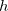✓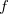HF ✓✓ROHF ✓✗ MP2 ✓✗ (V)OD ✓✗ (V)QCCD ✓✗ CIS (except RO) ✓✓CFMM ✓✗
Table 9.1: Gradients and Hessians currently available for geometry optimizations with maximum angular momentum types for analytical derivative calculations (for higher angular momentum, derivatives are computed numerically). Analytical Hessians are not yet available for meta-GGA functionals such as BMK and the M05 and M06 series.

Another consideration when trying to minimize the optimization time concerns the quality of the gradient and Hessian. A higher-quality Hessian (i.e., analytical versus approximate) will in many cases lead to faster convergence, in the sense of requiring fewer optimization steps. However, the construction of an analytical Hessian requires significant computational effort and may outweigh the advantage of fewer optimization cycles. Currently available analytical gradients and Hessians are summarized in Table 9.1.

Features of Q-Chem’s geometry and transition-state optimization capabilities include:

• Cartesian, Z-matrix or internal coordinate systems

• Eigenvector Following (EF) or GDIIS algorithms

• Constrained optimizations

• Equilibrium structure searches

• Transition structure searches

• Hessian-free characterization of stationary points

• Initial Hessian and Hessian update options

• Reaction pathways using intrinsic reaction coordinates (IRC)

• Optimization of minimum-energy crossing points (MECPs) along conical seams

## 9.1.2 Job Control

Obviously a level of theory, basis set, and starting molecular geometry must be specified to begin a geometry optimization or transition-structure search. These aspects are described elsewhere in this manual, and this section describes job-control variables specific to optimizations.

JOBTYPE
 Specifies the calculation.

TYPE:
 STRING

DEFAULT:
 Default is single-point, which should be changed to one of the following options.

OPTIONS:
 OPT Equilibrium structure optimization. TS Transition structure optimization. RPATH Intrinsic reaction path following.

RECOMMENDATION:
 Application-dependent.

GEOM_OPT_HESSIAN
 Determines the initial Hessian status.

TYPE:
 STRING

DEFAULT:
 DIAGONAL

OPTIONS:
 DIAGONAL Set up diagonal Hessian. READ Have exact or initial Hessian. Use as is if Cartesian, or transform if internals.

RECOMMENDATION:
 An accurate initial Hessian will improve the performance of the optimizer, but is expensive to compute.

GEOM_OPT_COORDS
 Controls the type of optimization coordinates.

TYPE:
 INTEGER

DEFAULT:1

OPTIONS:
 0 Optimize in Cartesian coordinates. 1 Generate and optimize in internal coordinates, if this fails abort.1 Generate and optimize in internal coordinates, if this fails at any stage of the optimization, switch to Cartesian and continue. 2 Optimize in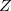-matrix coordinates, if this fails abort.2 Optimize in-matrix coordinates, if this fails during any stage of the optimization switch to Cartesians and continue.

RECOMMENDATION:
 Use the default; delocalized internals are more efficient.

TYPE:
 INTEGER

DEFAULT:
 300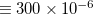tolerance on maximum gradient component.

OPTIONS:Integer value (tolerance =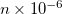).

RECOMMENDATION:
 Use the default. To converge GEOM_OPT_TOL_GRADIENT and one of GEOM_OPT_TOL_DISPLACEMENT and GEOM_OPT_TOL_ENERGY must be satisfied.

GEOM_OPT_TOL_DISPLACEMENT
 Convergence on maximum atomic displacement.

TYPE:
 INTEGER

DEFAULT:
 1200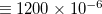tolerance on maximum atomic displacement.

OPTIONS:Integer value (tolerance =).

RECOMMENDATION:
 Use the default. To converge GEOM_OPT_TOL_GRADIENT and one of GEOM_OPT_TOL_DISPLACEMENT and GEOM_OPT_TOL_ENERGY must be satisfied.

GEOM_OPT_TOL_ENERGY
 Convergence on energy change of successive optimization cycles.

TYPE:
 INTEGER

DEFAULT:
 100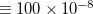tolerance on maximum (absolute) energy change.

OPTIONS:Integer value (tolerance = value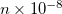).

RECOMMENDATION:
 Use the default. To converge GEOM_OPT_TOL_GRADIENT and one of GEOM_OPT_TOL_DISPLACEMENT and GEOM_OPT_TOL_ENERGY must be satisfied.

GEOM_OPT_MAX_CYCLES
 Maximum number of optimization cycles.

TYPE:
 INTEGER

DEFAULT:
 50

OPTIONS:User defined positive integer.

RECOMMENDATION:
 The default should be sufficient for most cases. Increase if the initial guess geometry is poor, or for systems with shallow potential wells.

GEOM_OPT_PRINT
 Controls the amount of Optimize print output.

TYPE:
 INTEGER

DEFAULT:
 3 Error messages, summary, warning, standard information and gradient print out.

OPTIONS:
 0 Error messages only. 1 Level 0 plus summary and warning print out. 2 Level 1 plus standard information. 3 Level 2 plus gradient print out. 4 Level 3 plus Hessian print out. 5 Level 4 plus iterative print out. 6 Level 5 plus internal generation print out. 7 Debug print out.

RECOMMENDATION:
 Use the default.

GEOM_OPT_SYMFLAG
 Controls the use of symmetry in Optimize.

TYPE:
 LOGICAL

DEFAULT:
 TRUE

OPTIONS:
 TRUE Make use of point group symmetry. FALSE Do not make use of point group symmetry.

RECOMMENDATION:
 Use the default.

GEOM_OPT_MODE
 Determines Hessian mode followed during a transition state search.

TYPE:
 INTEGER

DEFAULT:
 0

OPTIONS:
 0 Mode following off.Maximize along mode.

RECOMMENDATION:
 Use the default, for geometry optimizations.

GEOM_OPT_MAX_DIIS
 Controls maximum size of subspace for GDIIS.

TYPE:
 INTEGER

DEFAULT:
 0

OPTIONS:
 0 Do not use GDIIS. -1 Default size = min(NDEG, NATOMS, 4) NDEG = number of molecular degrees of freedom.Size specified by user.

RECOMMENDATION:
 Use the default or do not settoo large.

GEOM_OPT_DMAX
 Maximum allowed step size. Value supplied is multiplied by 10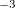.

TYPE:
 INTEGER

DEFAULT:
 300 = 0.3

OPTIONS:User-defined cutoff.

RECOMMENDATION:
 Use the default.

GEOM_OPT_UPDATE
 Controls the Hessian update algorithm.

TYPE:
 INTEGER

DEFAULT:
 -1

OPTIONS:
 -1 Use the default update algorithm. 0 Do not update the Hessian (not recommended). 1 Murtagh-Sargent update. 2 Powell update. 3 Powell/Murtagh-Sargent update (TS default). 4 BFGS update (OPT default). 5 BFGS with safeguards to ensure retention of positive definiteness (GDISS default).

RECOMMENDATION:
 Use the default.

GEOM_OPT_LINEAR_ANGLE
 Threshold for near linear bond angles (degrees).

TYPE:
 INTEGER

DEFAULT:
 165 degrees.

OPTIONS:User-defined level.

RECOMMENDATION:
 Use the default.

FDIFF_STEPSIZE
 Displacement used for calculating derivatives by finite difference.

TYPE:
 INTEGER

DEFAULT:
 100 Corresponding to 0.001 . For calculating second derivatives.

OPTIONS:Use a step size of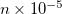.

RECOMMENDATION:
 Use the default except in cases where the potential surface is very flat, in which case a larger value should be used. See FDIFF_STEPSIZE_QFF for third and fourth derivatives.

Example 9.208  As outlined, the rate of convergence of the iterative optimization process is dependent on a number of factors, one of which is the use of an initial analytic Hessian. This is easily achieved by instructing Q-Chem to calculate an analytic Hessian and proceed then to determine the required critical point

$molecule 0 1 O H 1 oh H 1 oh 2 hoh oh = 1.1 hoh = 104$end

$rem JOBTYPE freq Calculate an analytic Hessian METHOD hf BASIS 6-31g(d)$end

$comment Now proceed with the Optimization making sure to read in the analytic Hessian (use other available information too).$end

@@@

$molecule read$end

$rem JOBTYPE opt METHOD hf BASIS 6-31g(d) SCF_GUESS read GEOM_OPT_HESSIAN read Have the initial Hessian$end


## 9.1.3 Hessian-Free Characterization of Stationary Points

Q-Chem allows the user to characterize the stationary point found by a geometry optimization or transition state search without performing a full analytical Hessian calculation, which is sometimes unavailable or computationally unaffordable. This is achieved via a finite difference Davidson procedure developed by Sharada et al. For a geometry optimization, it solves for the lowest eigenvalue of the Hessian (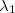) and checks if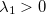(a negativeindicates a saddle point); for a TS search, it solves for the lowest two eigenvalues, and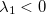and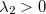indicate a transition state. The lowest eigenvectors of the updated P-RFO (approximate) Hessian at convergence are used as the initial guess for the Davidson solver.

The cost of this Hessian-free characterization method depends on the rate of convergence of the Davidson solver. For example, to characterize an energy minimum, it requires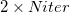total energy + gradient calculations, where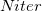is the number of iterations that the Davidson algorithm needs to converge, and “2" is for forward and backward displacements on each iteration. According to Ref. Sharada:2014, this method can be much more efficient than exact Hessian calculation for substantially large systems.

Note: At the moment, this method does not support QM/MM or systems with fixed atoms.

GEOM_OPT_CHARAC
 Use the finite difference Davidson method to characterize the resulting energy minimum/transition state.

TYPE:
 BOOLEAN

DEFAULT:
 FALSE

OPTIONS:
 FALSE do not characterize the resulting stationary point. TRUE perform a characterization of the stationary point.

RECOMMENDATION:
 Set it to TRUE when the character of a stationary point needs to be verified, especially for a transition structure.

GEOM_OPT_CHARAC_CONV
 Overide the built-in convergence criterion for the Davidson solver.

TYPE:
 INTEGER

DEFAULT:
 0 (use the built-in default value 10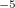)

OPTIONS:Set the convergence criterion to 10.

RECOMMENDATION:
 Use the default. If it fails to converge, consider loosening the criterion with caution.

Example 9.209  Geometry optimization of a triflate anion that converges to an eclipsed conformation, which is a first order saddle point. This is verified via the finite difference Davidson method by setting GEOM_OPT_CHARAC to TRUE.

$molecule -1 1 C 0.00000 -0.00078 0.98436 F -1.09414 -0.63166 1.47859 S 0.00000 0.00008 -0.94745 O 1.25831 -0.72597 -1.28972 O -1.25831 -0.72597 -1.28972 O 0.00000 1.45286 -1.28958 F 1.09414 -0.63166 1.47859 F 0.00000 1.26313 1.47663$end

$rem JOBTYPE opt METHOD BP86 GEOM_OPT_DMAX 50 BASIS 6-311+G* SCF_CONVERGENCE 8 THRESH 14 SYMMETRY FALSE SYM_IGNORE TRUE GEOM_OPT_TOL_DISPLACEMENT 10 GEOM_OPT_TOL_ENERGY 10 GEOM_OPT_TOL_GRADIENT 10 GEOM_OPT_CHARAC TRUE$end


Example 9.210  TS search for alanine dipeptide rearrangement reaction beginning with a guess structure converges correctly. The resulting TS structure is verified using the finite difference Davidson method.

$molecule 0 1 C 3.21659 -1.41022 -0.26053 C 2.16708 -0.35258 -0.59607 N 1.21359 -0.16703 0.41640 C 0.11616 0.82394 0.50964 C -1.19613 0.03585 0.74226 N -2.18193 -0.02502 -0.18081 C -3.43891 -0.74663 0.01614 O 2.19596 0.25708 -1.63440 C 0.11486 1.96253 -0.53088 O -1.29658 -0.59392 1.85462 H 3.25195 -2.14283 -1.08721 H 3.06369 -1.95423 0.67666 H 4.20892 -0.93714 -0.22851 H 1.24786 -0.78278 1.21013 H 0.25990 1.31404 1.47973 H -2.02230 0.38818 -1.10143 H -3.60706 -1.48647 -0.76756 H -4.29549 -0.06423 0.04327 H -3.36801 -1.25875 0.98106 H -0.68664 2.66864 -0.27269 H 0.01029 1.65112 -1.56461 H 1.06461 2.50818 -0.45885$end

$rem JOBTYPE freq EXCHANGE B3LYP BASIS 6-31G SCF_MAX_CYCLES 250 SYMMETRY false SYM_IGNORE true$end

@@@

$rem JOBTYPE ts SCF_GUESS read GEOM_OPT_DMAX 100 GEOM_OPT_MAX_CYCLES 1500 EXCHANGE B3LYP BASIS 6-31G MAX_SCF_CYCLES 250 GEOM_OPT_HESSIAN read SYMMETRY false SYM_IGNORE true GEOM_OPT_CHARAC true$end

$molecule read$end